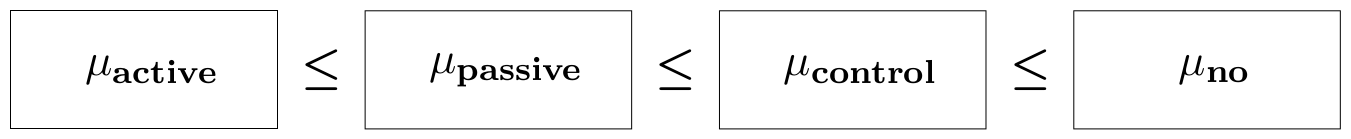## What is restriktor?

Restriktor allows for easy-to-use testing of linear equality and inequality restrictions about parameters and effects in linear models.

Many researchers have specific expectations about the relations between the parameters (e.g., means, regression coefficients). These expectations can often be expressed in terms of order/inequality constraints between the parameters. This is known as informative hypothesis testing. The one-sided t-test is the simplest example (e.g., $\mu_1 \geq$ 0 and $\mu_1 \leq \mu_2$). This readily extends to the multi-parameter setting, where more than one inequality constraint can be imposed on the parameters (e.g., $\mu_1 \leq \mu_2 \leq \mu_3$). Incorporating such prior knowledge in the hypothesis test has some benefits :

• testing the hypothesis more directly
• substantially more power
• no need for post-hoc tests
• no inflated type I error rate

The long-term goal of restriktor is to become the software tool for inequality and/or equality constraint estimation and testing, in a large variety of statistical models.

## Order-constrained means in an ANOVA layout

To get a first impression of how restriktor works in practice, consider the following example of order-constrained means. The dataset (Zelazo, Zelazo and Kolb, 1972) consists of ages (in months) at which an infant starts to walk alone from four different treatment groups (Active, Passive, Control, No). The assumption is that the walking exercises would not have a negative effect of increasing the mean age at which a child starts to walk. The figure below shows the model of our order-constrained hypothesis. The four groups are ordered by means of imposing inequality constraints (<, 'smaller than') between the group means.## to run this example, copy and paste the syntax below into R

library(restriktor)

## construct the constraint syntax. This can simply be done
## by using the factor level names (here Active, Passive,
## Control, No) preceded by the factor name (here Group) variable.
myConstraints <- ' GroupActive  < GroupPassive
GroupPassive < GroupControl
GroupControl < GroupNo '

## fit the unrestricted linear model. We removed the intercept (-1)
## such that the parameter estimates reflect the group means.
fit.ANOVA <- lm(Age ~ -1 + Group, data = ZelazoKolb1972)

## evaluate the informative hypothesis
iht(fit.ANOVA, constraints = myConstraints)


Restriktor: restricted hypothesis tests ( 19 residual degrees of freedom ):

Multiple R-squared remains 0.986

Constraint matrix:
GroupActive GroupControl GroupNo GroupPassive    op rhs active
1:          -1            0       0            1    >=   0     no
2:           0            1       0           -1    >=   0     no
3:           0           -1       1            0    >=   0     no

Overview of all available hypothesis tests:

Global test: H0: all parameters are restricted to be equal (==)
vs. HA: at least one inequality restriction is strictly true (>)
Test statistic: 6.4267,   p-value: 0.03085

Type A test: H0: all restrictions are equalities (==)
vs. HA: at least one inequality restriction is strictly true (>)
Test statistic: 6.4267,   p-value: 0.03085

Type B test: H0: all restrictions hold in the population
vs. HA: at least one restriction is violated
Test statistic: 0.0000,   p-value: 1

Type C test: H0: at least one restriction is false or active (==)
vs. HA: all restrictions are strictly true (>)
Test statistic: 0.3807,   p-value: 0.3538

Note: Type C test is based on a t-distribution (one-sided),
all other tests are based on a mixture of F-distributions.